# Fibonacci Word Problems I: Basic

Here and next week, we’ll look at a collection of word problems we have seen that involve the Fibonacci sequence or its relatives, sometimes on the surface, other times only deep down. The first set (here) are direct representations of Fibonacci, while the second set will be considerably deeper.

## A 20-foot walkway

The first, from 2002, is the most basic:

Laying a Brick Walkway

I have to build a walkway, 2 ft. by 20 ft., out of bricks that are 1 ft. by 2 ft. The bricks can lie vertically and horizontally but in no other direction.

How many different ways can I build this walkway?

I know there has to be a pattern, but I do not see it. Any help would be great.

Here is a representation of the problem: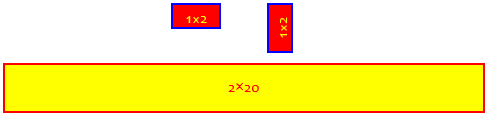Here are three of the many ways to lay the bricks: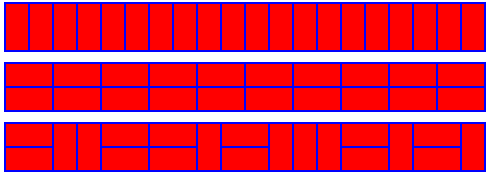On the surface, this is a combinatorics question, counting ways to do something; and we might approach it with permutations or combinations. In fact, we’ll be doing that for one of these problems later. But here, we’ll be finding a pattern, just as Dawn suggested.

Hi, Dawn.

When I don't see how to solve a problem like this, I start by playing with the ideas involved in the problem. That usually means simplifying the problem by working with smaller, easier numbers - actually, that's more or less what children's play is, isn't it? By trying things out in a smaller case, I get a feel for how it works. And in this case, that leads directly to a solution.

This is a very important step of problem solving, which I have elsewhere called the exploratory phase. Sometimes it only builds our understanding of the problem, but in others (as in inductive proofs) it becomes part of the solution.

How many ways can you make a 2-by-1 walkway (silly as it sounds!)? One, of course:

|
|

How about a 2-by-2 walkway? Two ways:

| |    ---
| |    ---

That is,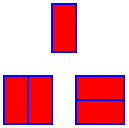How about 2-by-3? You can build on what you have already done: if the first brick you place is vertical (across the walk), you have 2 more feet to fill in, using one of the solutions for the 2-by-2. What happens if the first brick is horizontal (lengthwise)? See if you can use this to identify a pattern.

You will recognize a relationship to the Fibonacci sequence, which you can read about in the Dr. Math FAQ:

Golden Ratio, Fibonacci Sequence
http://mathforum.org/dr.math/faq/faq.golden.ratio.html

After a first vertical brick, we have a 2×2 to fill in, which we already know we can do in 2 ways: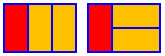After a first horizontal brick, we have to add another horizontal to match it, and then we have a 2×1 to fill in, which we already know we can do in 1 way: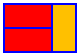So the number of ways to fill a 2×3 is $$1 + 2 = 3$$. Do you see Fibonacci hiding here?

Here is what we get if we repeat this procedure another time: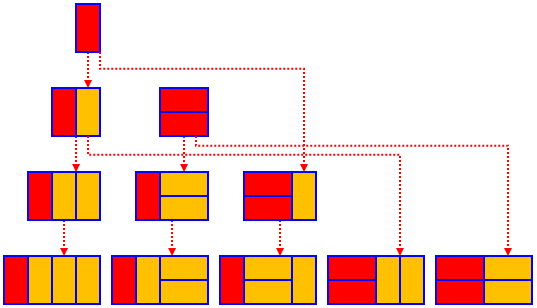For each row, we can either start with one vertical brick (red) and follow it (orange) with any possibility from the previous row, or start with two horizontal bricks and follow with any possibility from the second row up. This give the recursion $$A_N=A_{N-1}+A_{N-2}$$. Unlike the Fibonacci sequence, however, this starts with $$A_1=1, A_2=2$$. The answer will just be a renumbered Fibonacci sequence.

## A 15-foot walkway

The next question, from 2003, is very similar:

Fibonacci and Possible Tilings

I'm supposed to solve the following problem using Fibonacci's sequence:

You are going to pave a 15 ft by 2 ft walkway with
1 ft by 2 ft paving stones. How many possible ways
are there to pave the walkway?

However, I don't see how it relates to the problem. Can you help me get started?

I thought I just went to the 15th number in his sequence (because there are 15 stones) but I'm not quite sure why that would work, if it does.

This time, the problem was given together with a hint to use Fibonacci; but it’s not enough just to assume that is valid. We need to understand. Good for Ashley!

Doctor Floor answered, with an approach only slightly different than mine:

Hi, Ashley,

To get started, you can try to find out how you can pave a walkway that is N ft by 2 ft.

When N=1 it is easy, there is only one way.

When N=2 you can pave in two ways:
_ _      ___
| | |    |___|
|_|_| or |___|

I didn’t point out above that this is an example of a sometimes-surprising problem-solving technique: Solve a specific problem by generalizing it! We were asked for the specific case of 15 feet, but we make it doable by first generalizing it to N feet so we can find a formula. Then, in effect we work on that by induction, working step by step until we see what happens for all N.

We’ve seen already that the first two cases correspond to the second and third numbers in the Fibonacci sequence, $$F_1=1$$, $$F_2=1$$, $$F_3=2$$ – not the first two! So our final result will be that a walk of N feet can be made in $$F_{N+1}$$ ways.

To find number of ways of longer walkways, you have to realize that the smallest "units" you can add are
_          ___
1. | |     2. |___|
|_|        |___|

By these units the pavement becomes 1 ft or 2 ft longer respectively.

So whatever length we have, we have one way to lengthen it by one foot, or two ways to lengthen it by two feet.

To get a pavement of 3 ft by 2 ft you have to add to a 2 ft by 2 ft pavement unit (1), or to a 1 ft by 2 ft pavement unit (2). So the number of ways to make a pavement for N=3 is the sum of the numbers of ways for N=1 and N=2. Similarly for N=4 the number of ways is the sum of the numbers of ways for N=2 and N=3.

See the relation with Fibonacci's sequence?

This says essentially what I did: You can make a new walk by starting with a two-foot unit or a one-foot unit, and then build a walk you already know how to make. But when I read it, I initially saw it as working backward, and that can be interesting, too:

Suppose we imagine having found all the ways to make a walk of $$N-2$$ feet or a walk of $$N-1$$ feet first, and then lengthening each of the former with two horizontal blocks, and each of the latter with a single vertical block, leading to all possible walks of $$N$$ feet. (If we added two vertical blocks, we could have made the same walk by adding one vertical block to a walk of $$N-1$$ feet.)

This approach makes the recursion look just a little different: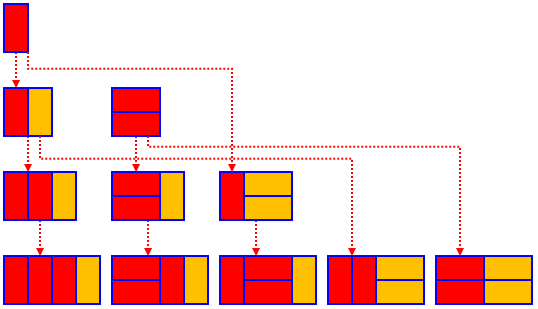So what’s the answer? Here is a table showing the answers to both the 20-foot and the 15-foot questions: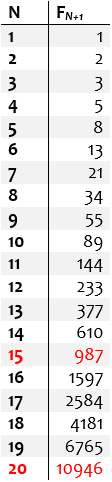Although we don’t have a direct formula for the answers, it took only a few additions to get them. If the numbers were larger, we might want to use the explicit formula we’ve seen elsewhere (with a calculator, since that requires powers of phi); we’ll have reason to do that for the next problem!

## Climbing 100 steps, 1 or 2 at a time

Our next problem is also from 2003:

Climbing 100 Steps

Find the number of ways in which you can climb 100 steps if you can go up 1 or 2 steps at a time.

I was thinking that n = 100 steps and there are two choices for each step you land on, but I wasn't sure how to break it down using 1 step and 2 steps at a time. I figured that there must be an even number of 1-steps and of course an even number of 2-steps to make 100, but I lost it there.

We can visualize the problem like this, showing one way to climb ten steps: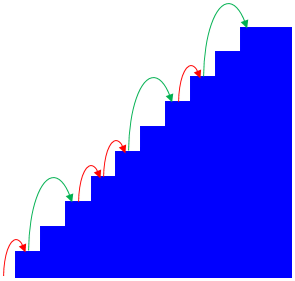We can picture that same example as a series of single- and double-steps in a top view, like this: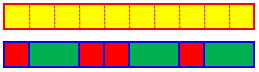Matt has some interesting thoughts. I think he meant that any number of “2-steps” will climb an even number of steps, so only the number of “1-steps” is restricted. And the idea of having two choices suggests he is thinking in terms of combinatorics.

### Lots of combinations

Doctor Robert answered first, taking that approach:

Start with an easier problem. Let's just have 10 steps. Then a table will show the number of 1-step and 2-step jumps needed.

1-step      2-step

10           0
8           1
6           2
4           3
2           4
0           5


That is, we can either take 10 single steps (that’s one way), or 8 single steps and 1 double step (arranged in various ways), and so on; so we can count 6 cases and add the resulting 6 numbers to get the total number of ways. And each of those numbers can be found as a combination:

Now the question is, "For each of these possibilities, how many ways can the different steps be arranged?" There's only one way to do the steps one at a time. There are nine ways to do eight one-step and one 2-step jumps. These numbers turn out to be C(10,0), C(9,1), respectively. For the next, the possibilities number C(8,2) or 28.  C(7,3)= 35  C(6,4) = 15  C(5,0) = 1. The total number of ways is therefore 1+9+28+35+15+1 = 89 ways for TEN steps.

Where do these come from? As an example, for the 8 singles and 1 double, we are talking about arranging the nine numbers 111111112 in all possible ways; this can be thought of as choosing which of 9 spaces to put the 2 into (and the rest will be 1’s). Similarly, for 6 singles and 2 doubles, we are arranging 11111122, so we need to choose which 2 of 8 spaces to put 2’s into. So the total number of ways in this case is $${{10}\choose 0}+{9\choose 1}+{8\choose 2}+{7\choose 3}+{6\choose 4}+{5\choose 5}=1+9+28+35+15+1=89$$

If you know about Pascal’s triangle, this forms an interesting pattern; we’ll later be seeing a proof that this pattern always yields … the Fibonacci numbers!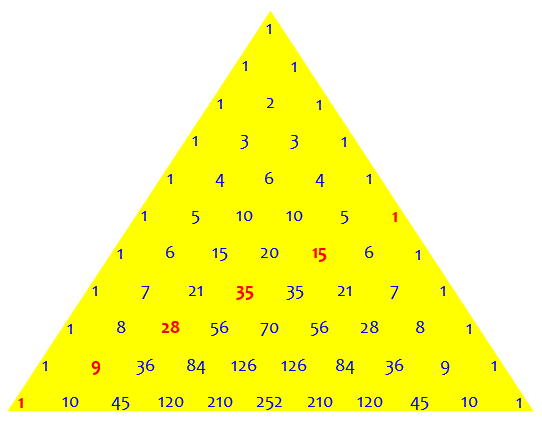(An interesting side problem would be: for N steps, how many combinations will we need to add?)

Good luck on the ONE HUNDRED steps.  There might be a clever way to find the sum without doing all the grunt work.

Yes, there is a clever way …

### Let Fibonacci do it

Doctor Douglas took it the next step:

Hi Matt,

Thanks for writing to the Math Forum.

I'd like to supplement Dr. Robert's answer. There is another way to approach this problem, which is to try to enumerate the number of ways to reach a given step.

step    ways to get there
----    -----------------
1            1            (has to be a 1-step)
2            2            (either a 2-step, or two 1-steps)
3            3            (either 1+1+1, 1+2, or 2+1)
4            5            (1+1+1+1, 1+2+1, 2+1+1, 1+1+2, 2+2)
5            8                Why?

Where Doctor Robert looked at a single middle-sized smaller example, Doctor Douglas is doing as we did in the previous problems, starting with the smallest examples and looking for a pattern. Both can be good ways to approach a problem; sometimes you need to try different ways to see what works best.

The reason is that in order to get to step 5, we must either get to step 3 (and take a 2-step), or get to step 4 (and take a 1-step).  Thus the number of ways to get to step 5 is the number of ways to get to step 3 plus the number of ways to get to step 4:

N(5) = N(4) + N(3)

The case of getting to step 3 and then taking a 1-step and then another 1-step is counted in the number of ways to get to step 4.

We can illustrate the recursion like this: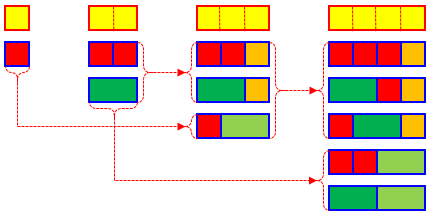Let's continue the table:

6           13
7           21
8           34
9           55
10           89          (so Dr. Robert's method is verified)
11          144

Do these numbers look familiar? If they do, they should - it is a Fibonacci sequence, and the answer to your question is the hundredth (or perhaps the 101st, depending on how you index things) Fibonacci number.

I'm sure you'll agree that this method is somewhat less work computationally than going through the entire combinatorial list.

Using the traditional indexing we have been using, the number of ways to get to the Nth step is $$F_{N+1}$$, so the answer to the question (the 100th step) is $$F_{101}$$.

This would still take 100 additions, which would be tiring (but easy on a spreadsheet … if it could hold the numbers!). Let’s use the shortcut formula we saw in The Golden Ratio and Fibonacci, by rounding: $$F_n = round\left(\frac{\phi^n}{\sqrt{5}}\right)\text{, for all }n \ge 0$$

Our answer is therefore $$F_{101} = round\left(\frac{\phi^{101}}{\sqrt{5}}\right)\\ = round\left(\frac{1,281,597,540,372,340,914,251}{2.236\ 067\ 977\ 499\ 789\ 696\ 409}\right)\\ = 573,147,844,013,817,084,101$$

My spreadsheet just says “5.73148E+20”, meaning $$5.73148\times 10^{20}$$, which agrees with my result from the Windows calculator.

Matt replied,

Thanks for the prompt replies; you guys are awesome. After seeing the numbers that were sent from that method, I figured I could use recurrence equations and get an answer a lot quicker for the 100 steps. It came out to be a messy answer but I'm almost 100% sure it's correct. I'm a mathematics major and your help will help me get a better grade on my final tomorrow no doubt. Thanks again.

Matt didn’t show his answer, but since he has learned about recurrence relations, there’s a good chance he did something like what we just did. Messy indeed!

## A phone chain: geometric, or Fibonacci?

I want to close with a problem from 1998 with two interpretations:

A Phone Chain

I'm trying to figure out if there is a formula for this problem.

There is a phone chain of people on a response team. When the first person gets a call, he calls two people, those two call two more, and each person calls two more. In all, 55 people need to be called. Figuring that each call takes one minute, estimate how long it will take to call 55 people. How long to call 100 people? How long to call n people?

We have already constructed a table showing how many people it takes to call 55 people, and how many people have been called in increments of 2 minutes. We have determined that in 2 minutes, 3 people have been called, in 4 minutes, 7 people have been called, in 6 minutes, 15 people have been called, etc. The first person calls 2, they call 4, they call 8, they call 16, etc. Is there any kind of formula to use to figure out how long it will take to call 100 people, to any number of people? Or is it just as easy to estimate from a chart if it is lengthened to cover the number of people who need to be called?

Thank you very much for your help.

The table they are making assumes a straightforward interpretation that leads to a geometric growth pattern, for which there is a simple formula. But what if we interpret it a little differently?

### As a geometric sequence

Hi, Leah. This is very interesting! I will answer your question first, but then I will point out that the problem is a little trickier than it looks at first.

It is easy to find a pattern in the numbers if we start with your second list, the number of NEW people called every 2 minutes: 2, 4, 8, 16, .... Each number in the list is twice the number right before it: 4 = 2 * 2, 8 = 2 * 4, etc. You probably figured this out already: if each person calls 2 people, then the number of people called is twice as many as the number called two minutes ago.

These numbers are the POWERS OF 2. The second number is 2 * 2, which is 2 squared or 2^2.

So the number of new people called in the nth pair of minutes is $$2^n$$.

Now look at the total number of people called: 3, 7, 15, etc. Do you notice that each number in the first list is one less than the next number in the second list? This is the pattern we see:

1 + 2             =  3 = 2^2 - 1
1 + 2 + 2^2       =  7 = 2^3 - 1
1 + 2 + 2^2 + 2^3 = 15 = 2^4 - 1
etc.

You can see why this is true if you think about what happens, for instance, when you add 2^3 to 2^3 - 1.

This is the sum of a geometric series. Our table, up to at least 55 people called, looks like this: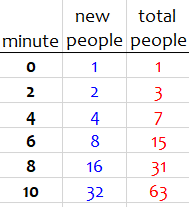To find the time required to call n people, we would solve the equation $$2^{t/2+1}-1=n$$, using logarithms, and round.

But though the problem is solvable in this interpretation (at least if Leah has learned about logs), there’s something we haven’t taken into account:

### As a Fibonacci sequence

Now let's take another look at the problem. I think what the problem says is a little different from what you thought, and this little difference changes the answer quite a bit.

You were thinking that each person calls 2 people in 2 minutes, and then after the 2 minutes, those 2 people each call 2 more people. But I don't think that is quite what will happen. The first person calls one person FIRST, taking 1 minute. Then while the first person makes a second phone call, the first person she called is ALREADY calling someone else! (Why wait a minute?) It will look like this:

Calls       Total
per minute  calls
----------  -----
1               1        1
+----------------+
|                |
2                |       1        2
+----------+           |
|          |           |
3          |           4       2        4
+------+      |       +------+
|      |      |       |      |
5      |      6       7      |    3        7
+---+    |    +---+   +---+    |
|   |    |    |   |   |   |    |
8   |    9   10   |  11   |   12    5       12

Person 1 calls person 2 first, then person 4, but in the meantime person 2 is already calling person 3. Do you see how it goes?

You may already have observed that the numbers in the “calls per minute” column look like the Fibonacci sequence; so the “total calls” column is the sum of the Fibonacci series, which we looked at in Generalizing and Summing the Fibonacci Sequence.

I have listed how many people are called each MINUTE (not every 2 minutes). If you want to compare it with what you found, remember to take this difference into account.

See if you can figure out how the pattern continues. If you have heard of the Fibonacci numbers, you'll get the idea. Then you can keep building the list, adding up as you go until the sum of all the numbers in the list reaches 55.

He left open the question of proving that this is what it looks like, and I’ll leave it open too. But assuming it is (and it is), the formula for the total calls after n minutes is $$F_{n+2}-1$$. Here is a table: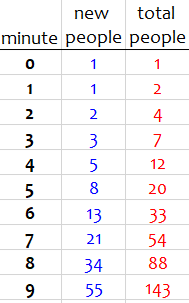Where before it took 9-10 minutes to get to 55 people, now it takes only 8 (and just missed 7).

There is a problem, though: There is no easy way to invert the formula and find the number of minutes to reach n people. So I can’t say for sure which interpretation the teacher intended!

Next week, we’ll look at a couple much more challenging twists on Fibonacci problems.

### 2 thoughts on “Fibonacci Word Problems I: Basic”

This site uses Akismet to reduce spam. Learn how your comment data is processed.